# Q3 Party Planner: Formula

13. Dig the Data

This demo uses Google Sheets. This works the same as in Excel except where noted in the steps below.

## Introduction

You have now created and formatted your basic spreadsheet. One of the really useful functions of a spreadsheet is its ability to do calculations. It can do much more than a calculator can do if you know how to create a formula. All formulas start with an equal sign. Let's calculate how many people will be coming to your party!

## I Can Statement

• calculate numbers using a spreadsheet

## Key Vocabulary

• Average: The function of finding the average value, or arithmetic mean, of values in a selected range of cells. (=average)
• Count: The function of totaling the number of cells in a selected range that contain a specific type of data. (=count)
• Max: The function of finding the largest or maximum number in a given list of values. (=max)
• Min: The function of finding the smallest or minimum number in a given list of values. (=min)

Play the Vocabulary Game below to practice the Key Vocabulary

You can change the Quiz mode to Match, Test, Learn, Flash Cards, Spell using the selection list at the bottom right of the activity that says "Choose a Study Mode." Direct Link

## STEPS for Using a Formula to Add a Column

Using directions found in Using a Formula to Add a Column PDF, complete the following:

Step 1 - Create an addition formula to add up the total invitees start with the = symbol, followed by SUM and an open parent (then drag down across the cells and a closed parents ) and hit enter.  Your formula should look like =SUM(B3:B7) where the : indicates including all the cells between B3 and B7.

Step 2 - Create an addition formula to find total attending in the next column in a similar way. Note: after you enter the formula, click on Return or Enter, and the total will appear instead of the formula.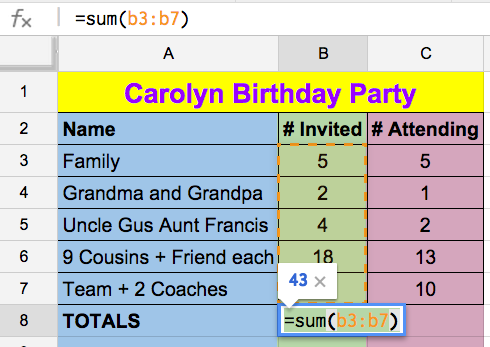Step 3 - Make sure that your formulas work.

Step 4 - How to Show Formulas:

• Google Sheets: Select the View menu and Show formulas. Then take a screenshot.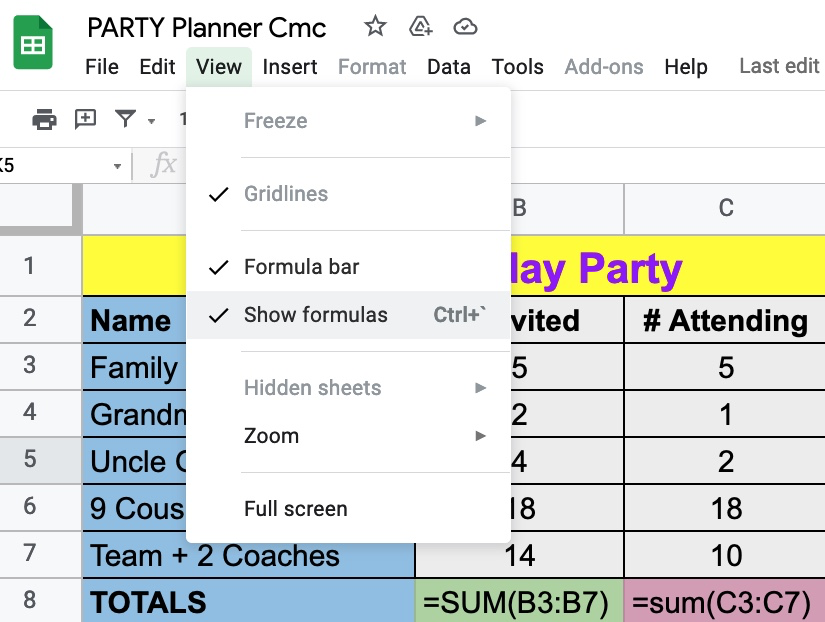• Microsoft Excel: Select the Formulas Menu and select Show Formulas.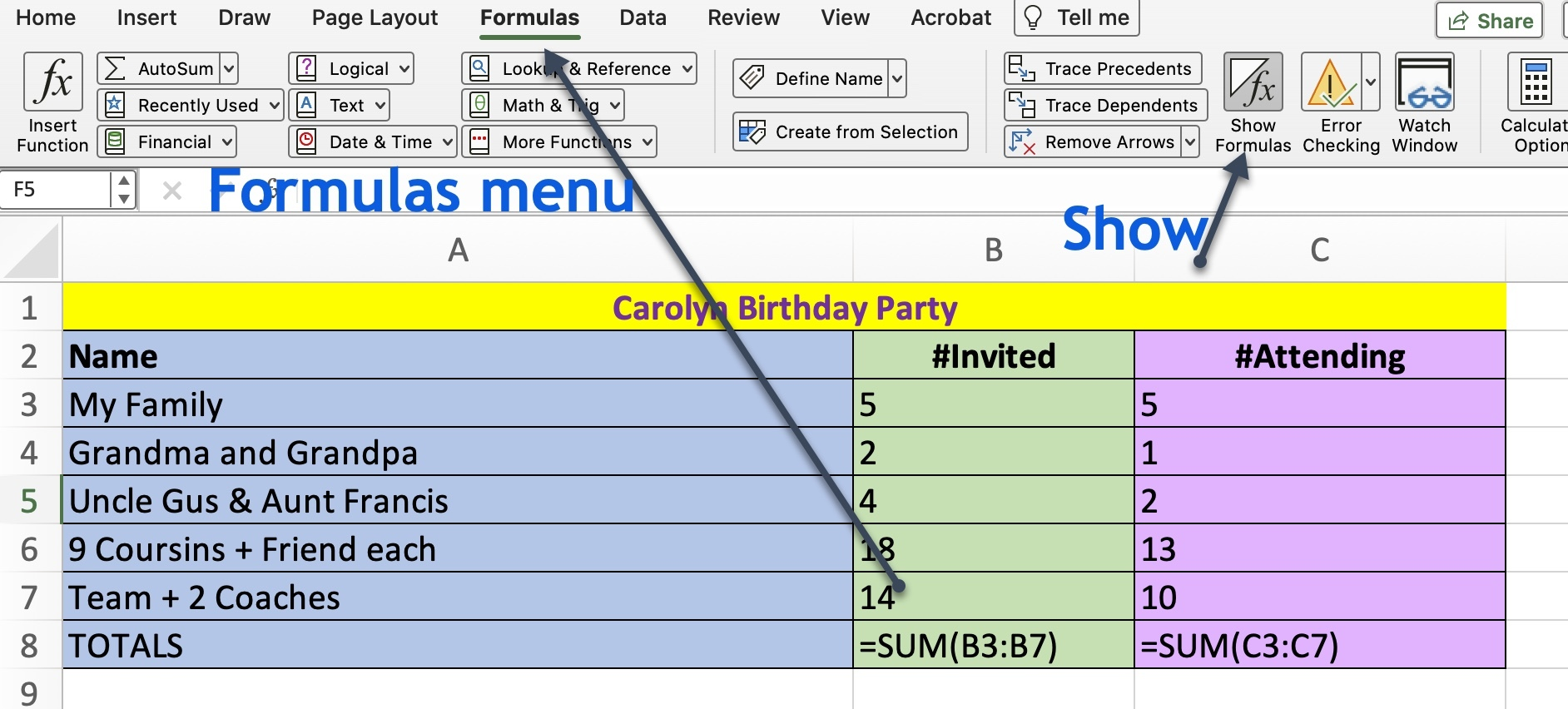Step 5 - Save your screenshot with one of the formulas showing.

Step 7 - Check with your teacher to see how they want you to submit or share this document.

Google has an Explore feature (2021) where you can ask questions about your data. It can show graphs it suggests to you, total things up, find the largest or smallest, and show you the formula or a chart.

The Explore icon is found on the bottom right area of your sheet. It looks like this: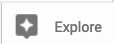Ask a question in the box labeled "Ask about this data"

Here are some examples using the Party Planner spreadsheet showing the Questions asked and screenshots of the answers.

 Question Typed into Explore: Results Question: What is the total # Attending?   Google explore shows an answer of 34   NOTE: You must use the name of something you are asking about such at #Attending that is in a cell.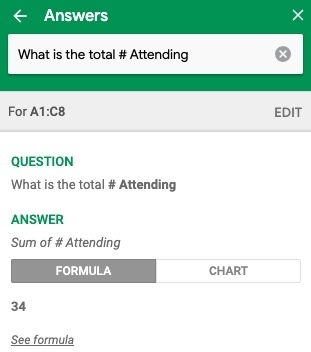Action: Then Click on the Formula to see the formula   Google answer shows the formula to get the sum of the #Attending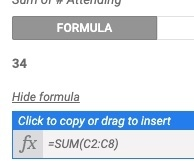Question: Names of the #Invited? Google answer shows the names and number invited for each named group. Try clicking on the Formula and then the Chart to discover more.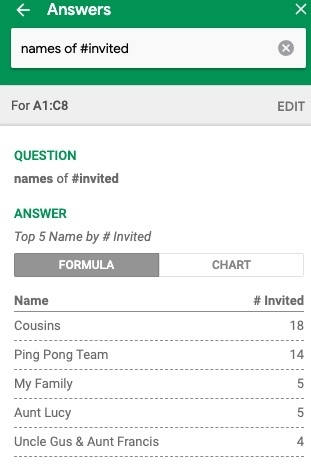Microsoft Excel

Check off this Quest on the 21t4s roadmap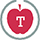13.Q3 Teacher Guide

MITECS  Michigan Integrated Technology Competencies for Students, and

ISTE Standards for Students

1. Empowered Learner
d. Understand the fundamental concepts of technology operations, demonstrate the ability to choose, use and troubleshoot current technologies and are able to transfer their knowledge to explore emerging technologies

3. Knowledge Constructor
c. Curate information from digital resources using a variety of tools and methods to create collections of artifacts that demonstrate meaningful connections or conclusions

5. Computational Thinker
b. Collect data or identify relevant data sets, use digital tools to analyze them, and represent data in various ways to facilitate problem-solving and decision-making
d. Understands how automation works and use algorithmic thinking to develop a sequence of steps to create and test automated solutions